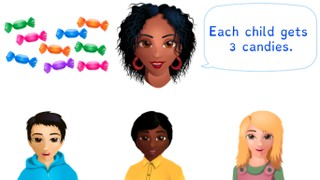Dividing a number to 100

# Dividing a number to 100

Students learn to divide numbers to 100.8,000 schools use Gynzy92,000 teachers use Gynzy1,600,000 students use Gynzy

## General

The students learn to divide a number to 100.

## Standards

CCSS.Math.Content.4.NBT.B.6

## Learning objective

Students will be able to divide numbers to 100 without a remainder.

## Introduction

The students solve the division problems.

## Instruction

Explain that in division problems with a number to 100 you first see which number from the table of the divisor is the closest to but less than the dividend. For the problem 36 ÷ 3 that is 10 × 3 = 30. Subtract the 30 from the 36. You now have 6 left over. 2 × 3 = 6. Next you do 6 - 6 = 0. Now you add the 10 and 2 together for your answer (12). Have the students solve the division problems on their own. Explain that for the problem 64 ÷ 16 you are going to see how many times you can subtract 16 from 64. You can also flip the problem around and see how many times you have to multiply 16 to get to 64. Show in steps that you get to 0 in 4 steps. So the answer is 4. Have the students solve the next couple division problems on their own. For the story problem, explain that you first take the problem from the story. So 93 ÷ 3. Next you look in the table of 3. If you know that 3 × 3 = 9, then you also know that 30 × 3 = 90. Now you still have 3 left over. 1 × 3 = 3. Your answer is now 30 + 1 = 31. Have the students solve the next two story problems.

Check if the students can divide a number to 100, by asking the following question:
- What steps do you follow to solve the problem 56 ÷ 14?

## Quiz

Students test their understanding of dividing a number to 100 through ten exercises. Some exercises are abstract division problems, and others are story problems.

## Closing

Discuss once again the importance of being able to divide a number to 100. As a closing activity you have the students drag the correct answers to their problems. Next they can solve the problem that is left over and write down the answer.

## Teaching tips

Have students that have difficulty with dividing with a number to 100 first practice with the division tables.

### The online teaching platform for interactive whiteboards and displays in schools

• Save time building lessons

• Manage the classroom more efficiently

• Increase student engagement